# Mass Transfer Effect on a Rotating MHD Transient Flow of Liquid Lead Through a Porous Medium in Presence of Hall and Ion Slip Current with Radiation

Mass Transfer Effect on a Rotating MHD Transient Flow of Liquid Lead Through a Porous Medium in Presence of Hall and Ion Slip Current with Radiation
Department of Mathematics, Gauhati University, Guwahati-14, Assam 781014, India

Corresponding Author Email:
parasharpranabjyoti@gmail.com
Page:
134-141
|
DOI:
https://doi.org/10.18280/mmep.080117
9 January 2020
|
Accepted:
14 November 2020
|
Published:
28 February 2021
| Citation

OPEN ACCESS

Abstract:

A problem of unsteady MHD convective flow of liquid lead through an impulsively started semi infinite vertical porous plate in presence of a transversely applied uniform magnetic field under the effects of Hall current, ion slip current and chemical reaction is investigated. The fluid is considered to be incompressible while the magnetic Reynolds number is assumed to be very small. An exact solution to the flow model is obtained adopting Laplace Transform Technique in closed form. The effects of the relevant physical parameters on the velocity field, temperature field and concentration field are displayed graphically and the effects on skin friction, Nusselt number and Sherwood number are presented in tabular form.

Keywords:

hall current, ion slip current, heat and mass transfer

1. Introduction

Hall Effect is not considered in the generalized Ohm’s law. But in a situation when the applied magnetic field is very strong, the inclusion of Hall Effect in the generalized Ohm’s law is necessary. It is the deflection of the electric charges due to the electromagnetic force and so a new component of the current density vector needs to be considered. So the equation of the electric current density is derived from the diffusion velocity of electrons so as to include the Hall current rather than considering only the electromagnetic forces. Current research works in MHD trend towards a strong magnetic field which is to be addressed by considering Hall current. Cowling  first emphasized on the modification of Ohm’s law to include Hall current when dealing with a strong magnetic field. Pop  analyzed the MHD convection flow near an accelerated plate incorporating the effects of Hall current. The importance of Hall Effect in the Hartmann channel flow has been indicated by Cramer and Pai . Ahmed and Sarmah  have studied the unsteady MHD rotational flow past an impulsively started infinite horizontal porous plate with Hall current. Seth et al.  have investigated the effects of Hall current and rotation on the free convective MHD flow past a suddenly started moving vertical plate. Seth et al.  later studied the Hall current effects on unsteady MHD rotating natural convection free stream flow with exponential acceleration past a vertical plate.

Usually diffusion velocity of ions is neglected as it is much smaller than that of electrons when considering current density. But a strong electromagnetic force demands to consider the diffusion velocity of ions as well. The ion slip current is due to the diffusion velocity of ions. Mohanty  studied the hydromagnetic Rayleigh problem with Hall Effect. Soundalgekar et al.  have studied the Hall and ion slip effects on the MHD Couette flow with consideration of heat transfer phenomenon. Hall and ion slip current effects on MHD convective three dimensional flow of liquid metal with rotation was studied by Ghosh et al. . Sheikh and Ahmed  have studied the effects of radiation, Hall current and ion slip current on the rotating MHD transient flow of liquid metal through a porous medium.

Study of combined heat and mass transfer has its own relevance since many industrial, scientific processes and natural events are associated with this phenomenon. Mass transfer process does have the impact on heat transfer. Diffusion of Chemical species affects the flow properties of the fluid also. Eckert and Drake  and some other researchers made significant contributions to the studies of heat and mass transfer problems. Because of its increasing relevance, further researches were carried out in the following years by many authors. Some names in this regard are Raptis et al. , Acharya et al. , Reddy et al. , Lavanya and Leela Ratnam .

Our objective in the present work is to study the mass transfer phenomenon on the unsteady MHD rotating convective flow under the effects of Hall and ion slip current in presence of thermal radiation.

2. Mathematical Analysis

We consider the transient convective motion of an incompressible, viscous, partially ionized fluid past a semi-infinite plate through a porous medium. A rectangular Cartesian co-ordinate system is introduced so that the vertically upward direction along the plate becomes the X' axis, the perpendicular to the X' axis in the plate’s plane becomes the Y' axis and Z' axis is along the normal to the X'Y' plane directed towards the fluid region. The flow geometry is given below in Figure 1.

It is assumed that the fluid as well as the plate is rotating about the Z' axis with uniform angular velocity $\vec{\omega}$. A strong magnetic field $\vec{B}$ of intensity B0 is applied to the system in the positive Z' direction that is transversely to the plane of the flow. At time t'=0, the plate temperature is same with the free stream fluid temperature $T_{\infty}^{\prime}$ and concentration at the plate is assumed to be equal with the free stream fluid concentration $C_{\infty}^{\prime}$. At time t'>0, due to the application of an impulsive force, the plate velocity, temperature at the plate and the concentration at the plate rise suddenly to $U_{0}, T_{w}^{\prime}$ and $C_{w}^{\prime}$ respectively which remain constant throughout the motion.

## 1.pngFigure 1. Physical model of the problem

The magnetic Reynolds number in the problem is assumed to be very small. Due to this assumption, the induced magnetic field is ignored in this investigation. But in view of the relative motion of the particles in the partially ionized fluid, Hall current is considered in this study. With magnetic field $\vec{B}$ and electrical field $\vec{E}$ acting in directions perpendicular to each other, the electromagnetic Lorentz force acts in a direction perpendicular to both $\vec{B}$ and $\vec{E}$, causing the charged particles to drift in that direction. Consequently, the current density vector $\vec{J}$ will have a component in that direction, known as the Hall current. Further, the intensity B0 of the applied magnetic field is strong enough to consider the diffusion velocity of the ions, causing the ion-slip current. Taking into account the Hall current and ion-slip effect, following Sherman and Sutton , the electric current density vector $\vec{J}=\left(J_{x^{\prime}}, J_{y^{\prime}} J_{z^{\prime}}\right)$ is taken as:

$J_{x^{\prime}}=\left[\alpha_{1}\left(E_{x^{\prime}}+B_{0} V^{\prime}\right)-\beta_{1}\left(E_{y^{\prime}}-B_{0} U^{\prime}\right)\right] \sigma$

$J_{y^{\prime}}=\left[\alpha_{1}\left(E_{y^{\prime}}-B_{0} U^{\prime}\right)+\beta_{1}\left(E_{x^{\prime}}+B_{0} V^{\prime}\right)\right] \sigma$

$J_{z^{\prime}}=0\left(\begin{array}{l}\text { since the plate is taken electrically } \\ \text { non-conducting }\end{array}\right)$

where, $\alpha_{1}=\frac{1+\beta_{i} \beta_{e}}{\left(1+\beta_{i} \beta_{e}\right)^{2}+\left(\beta_{e}\right)^{2}}$ and $\beta_{1}=\frac{\beta_{e}}{\left(1+\beta_{i} \beta_{e}\right)^{2}+\left(\beta_{e}\right)^{2}}$.

The porous medium is assumed to be homogeneous and isotropic. This assumption allows us to use a single permeability for the porous medium in the simulation of hydrolic conductivity. The pressure gradient across the fluid medium is defined on the basis of Darcian drag force model as: $\nabla p=-\frac{\mu \vec{q}}{K_{p}^{*}}$.

Due to the above assumptions, the Equations governing the flow and the heat and mass transfer characteristics can now be written as (neglecting the convective acceleration terms):

$\rho\left(\frac{\partial U^{\prime}}{\partial t^{\prime}}-2 \omega V^{\prime}\right)=\mu \frac{\partial^{2} U^{\prime}}{\partial z^{\prime 2}}-\frac{\partial p}{\partial x^{\prime}}+J_{y^{\prime}} B_{0}-\frac{\mu U^{\prime}}{K_{p}^{*}}-\rho g$    (1)

$\rho\left(\frac{\partial V^{\prime}}{\partial t^{\prime}}+2 \omega U^{\prime}\right)=\mu \frac{\partial^{2} V^{\prime}}{\partial z^{\prime 2}}-\frac{\partial p}{\partial y^{\prime}}-J_{x^{\prime}} B_{0}-\frac{\mu V^{\prime}}{K_{p}^{*}}$    (2)

$\frac{\partial T^{\prime}}{\partial t^{\prime}}=\frac{\kappa}{\rho C_{p}} \frac{\partial^{2} T^{\prime}}{\partial z^{\prime 2}}-\frac{4 I}{\rho C_{p}}\left(T^{\prime}-T_{\infty}{ }^{\prime}\right)$    (3)

$\frac{{\partial C}^{\prime}}{{\partial}t^{\prime}}=D_{M} \frac{\partial^{2} C^{\prime}}{\partial z^{\prime 2}}+K_{1}\left({C^{\prime}}_{\infty}-{C^{\prime}}\right)$    (4)

In Eq. (3), $I=\int_{0}^{\infty}\left(a_{\lambda}\right)_{w}\left(\frac{\partial e_{\lambda h}}{\partial T^{\prime}}\right) d \lambda$.

In the free stream, U'=V'=0, $\rho=\rho_{\infty}$. Thus Eqns. (1) and (2) take the form:

$\frac{\partial p}{\partial x^{\prime}}=\left(\alpha_{1} E_{y^{\prime}}+\beta_{1} E_{x^{\prime}}\right) B_{0} \sigma-\rho_{\infty} g$    (5)

$\frac{\partial p}{\partial y^{\prime}}=\left(\beta_{1} E_{y^{\prime}}-\alpha_{1} E_{x^{\prime}}\right) B_{0} \sigma$    (6)

Implementation of the equation of state according to Bousinnesq approximation gives:

$\rho_{\infty}=\rho+\rho \beta\left(T^{\prime}-T_{\infty}^{\prime}\right)+\rho \beta^{*}\left(C^{\prime}-C_{\infty}^{\prime}\right)$    (7)

Due to (5), (6) and (7); Eqns. (1) and (2) now can be written as:

\begin{aligned} \frac{\partial U^{\prime}}{\partial t^{\prime}}-2 \omega V^{\prime} &=v \frac{\partial^{2} U^{\prime}}{\partial z^{\prime 2}}+\frac{B_{0}^{2} \sigma}{\rho}\left(\beta_{1} V^{\prime}-\alpha_{1} U^{\prime}\right)-\frac{v U^{\prime}}{K_{p}^{*}} \\ &+\beta g\left(T^{\prime}-T_{\infty}^{\prime}\right)+\beta^{*} g\left(C^{\prime}-C_{\infty}^{\prime}\right) \end{aligned}    (8)

$\frac{\partial V^{\prime}}{\partial t^{\prime}}+2 \omega U^{\prime}=v \frac{\partial^{2} V^{\prime}}{\partial z^{\prime 2}}-\frac{B_{0}^{2} \sigma}{\rho}\left(\beta_{1} U^{\prime}+\alpha_{1} V^{\prime}\right)-\frac{v V^{\prime}}{K_{p}^{\prime}}$    (9)

Now introducing two complex variables q'=U'+iV' and $\alpha_{0}=\alpha_{1}+i \beta_{1}$, momentum conservation equations represented by (8) and (9) are combined together to transform into a single equation given by (10):

\begin{aligned} \frac{\partial q^{\prime}}{\partial t^{\prime}}+2 i \omega q^{\prime}=v \frac{\partial^{2} q^{\prime}}{\partial z^{\prime 2}}-\frac{B_{0}^{2} \sigma \alpha_{0}}{\rho} q^{\prime}-& \frac{v q^{\prime}}{K_{p}^{*}}+\beta g\left(T^{\prime}-T_{\infty}^{\prime}\right) \\ &+\beta^{*} g\left(C^{\prime}-C_{\infty}^{\prime}\right) \end{aligned}    (10)

The initial and boundary conditions for the momentum conservation Eq. (10), energy conservation Eq. (3) and the species continuity Eq. (4) are assumed as:

$\left.\begin{array}{l}t^{\prime} \leq 0, z^{\prime}=0: q^{\prime}=0\left(U^{\prime}=0, V^{\prime}=0\right), T^{\prime}=T_{\infty}^{\prime}, C^{\prime}=C_{\infty}^{\prime} \\ t^{\prime}>0, z^{\prime}=0: q^{\prime}=U_{0}\left(U^{\prime}=U_{0}, V^{\prime}=0\right), T^{\prime}=T_{w}^{\prime}, C^{\prime}=C_{w}^{\prime} \\ t^{\prime}>0, z^{\prime} \rightarrow \infty: q^{\prime} \rightarrow 0\left(U^{\prime} \rightarrow 0, V^{\prime} \rightarrow 0\right), T^{\prime} \rightarrow T_{\infty}^{\prime}, C^{\prime} \rightarrow C_{\infty}^{\prime}\end{array}\right\}$    (11)

For the sake of idealization of the problem, the following non-dimensional quantities are introduced:

$\left.\begin{array}{l}U=\frac{U^{\prime}}{U_{0}}, V=\frac{V^{\prime}}{U_{0}}, q=\frac{q^{\prime}}{U_{0}}, t=\frac{t^{\prime} U_{0}^{2}}{v}, z=\frac{z^{\prime} U_{0}}{v} \\ \theta=\frac{T^{\prime}-T_{\infty}^{\prime}}{T_{w}^{\prime}-T_{\infty}^{\prime}}, \phi=\frac{C^{\prime}-C_{\infty}^{\prime}}{C_{w}^{\prime}-C_{\infty}^{\prime}} \\ \mathrm{Gr}=\frac{g \beta v\left(T^{\prime}-T_{\infty}^{\prime}\right)}{U_{0}^{3}}, \mathrm{Gm}=\frac{g \beta^{\prime} v\left(C^{\prime}-C_{\infty}^{\prime}\right)}{U_{0}^{3}} \\ E r=\frac{\omega v}{U_{0}^{2}}, N m^{2}=\frac{B_{0}^{2} v \sigma}{\rho U_{0}^{2}}, \mathrm{Da}=\frac{K_{p}^{*}}{L^{2}}, \mathrm{Re}=\frac{U_{0} L}{v} \\ N=\frac{4 I v}{\rho C_{p} U_{0}^{2}}, \operatorname{Pr}=\frac{\mu C_{p}}{\kappa}, K=\frac{K_{1} v}{U_{0}^{2}}, \mathrm{Sc}=\frac{v}{D_{M}}\end{array}\right\}$    (12)

By the aid of (12), Eqns. (10), (3) and (4) are idealized as follow:

$\frac{\partial q}{\partial t}=\frac{\partial^{2} q}{\partial z^{2}}+\operatorname{Gr} \theta+\operatorname{Gm} \phi-a q$    (13)

$\frac{\partial \theta}{\partial t}=\frac{1}{\operatorname{Pr}} \frac{\partial^{2} \theta}{\partial z^{2}}-N \theta$    (14)

$\frac{\partial \phi}{\partial t}=\frac{1}{\mathrm{Sc}} \frac{\partial^{2} \phi}{\partial z^{2}}-K \phi$    (15)

Corresponding idealized initial and boundary conditions are:

$\left.\begin{array}{l}t \leq 0, z=0: q=0, \theta=0, \phi=0 \\ t>0, z=0: q=1, \theta=1, \phi=1 \\ t>0, z \rightarrow \infty: q \rightarrow 0, \theta \rightarrow 0, \phi \rightarrow 0\end{array}\right\}$    (16)

3. Method of Solution

We have adopted Laplace transformation technique in closed form to find solutions for velocity field, temperature field and concentration field. Laplace transforms of the governing Eqns. (13), (14), (15) and the initial and boundary conditions (16) are as follow:

$\frac{d^{2} \bar{q}}{d z^{2}}-(s+a) \bar{q}=-\operatorname{Gr} \bar{\theta}-\operatorname{Gm} \bar{\phi}$    (17)

$\frac{d^{2} \bar{\theta}}{d z^{2}}-\operatorname{Pr}(s+N) \bar{\theta}=0$    (18)

$\frac{d^{2} \bar{\phi}}{d z^{2}}-\mathrm{Sc}(s+K) \bar{\phi}=0$    (19)

$\left.\begin{array}{l}t \leq 0, z=0: \bar{q}=0, \bar{\theta}=0, \bar{\phi}=0 \\ t>0, z=0: \bar{q}=\frac{1}{s}, \bar{\theta}=\frac{1}{s}, \bar{\phi}=\frac{1}{s} \\ t>0, z \rightarrow \infty: \bar{q} \rightarrow 0, \bar{\theta} \rightarrow 0, \bar{\phi} \rightarrow 0\end{array}\right\}$    (20)

Here $\bar{f}(z, s)$ denotes Laplace transform of f(z,t).

Solving (17), (18) and (19) subject to (20), the following solutions are obtained:

\begin{aligned} \bar{q}=&\left[\frac{1}{s}+\frac{1}{s}\left(\frac{B_{1}}{s-D_{1}}+\frac{E_{1}}{s-F_{1}}\right)\right] e^{-z \sqrt{s+a}} \\ &-\frac{1}{s}\left[\frac{B_{1} e^{-z \sqrt{\operatorname{Pr}(s+N)}}}{s-D_{1}}+\frac{E_{1} e^{-z \sqrt{\operatorname{Sc}(s+K)}}}{s-F_{1}}\right] \end{aligned}    (21)

$\bar{\theta}=\frac{1}{s} e^{-z \sqrt{\operatorname{Pr}(s+N)}}$    (22)

$\bar{\phi}=\frac{1}{s} e^{-z \sqrt{\operatorname{Sc}(s+K)}}$    (23)

Finally, inverse Laplace transformation is applied on Eqns. (21), (22) and (23) leading to the velocity, temperature and concentration distributions of this flow problem represented by Eqns. (24), (25) and (26) respectively as follow:

\begin{aligned} q=&\left(1-\frac{B_{1}}{D_{1}}-\frac{E_{1}}{F_{1}}\right) \psi(1, a, z, t)+\frac{B_{1}}{D_{1}} \psi(\operatorname{Pr}, N, z, t)+\\ & \frac{E_{1}}{F_{1}} \psi(\mathrm{Sc}, K, z, t)+\\ & \frac{B_{1}}{D_{1}} e^{D_{1} t}\left\{\psi\left(1, a+D_{1}, z, t\right)-\psi\left(\operatorname{Pr}, N+D_{1}, z, t\right)\right\}+\\ & \frac{E_{1}}{F_{1}} e^{F_{1} t}\left\{\psi\left(1, a+F_{1}, z, t\right)-\psi\left(\mathrm{Sc}, K+F_{1}, z, t\right)\right\} \end{aligned}    (24)

$\theta=\psi(\operatorname{Pr}, N, z, t)$    (25)

$\phi=\psi(\mathrm{Sc}, K, z, t)$    (26)

4. Skin Friction

Skin friction is defined by Newton’s law of viscosity. The coefficient of skin friction, Cf at the plate is computed as follows:

\begin{aligned} C_{f} &\left.=-\frac{\partial q}{\partial z}\right]_{z=0} \\ &=\left(1-\frac{B_{1}}{D_{1}}-\frac{E_{1}}{F_{1}}\right) \psi^{\prime}(1, a, t)+\frac{B_{1}}{D_{1}} \psi^{\prime}(\operatorname{Pr}, N, t)+\\ & \frac{E_{1}}{F_{1}} \psi^{\prime}(\mathrm{Sc}, K, t)+\\ & \frac{B_{1}}{D_{1}} e^{D_{1} t}\left\{\psi^{\prime}\left(1, a+D_{1}, t\right)-\psi^{\prime}\left(\operatorname{Pr}, N+D_{1}, t\right)\right\}+\\ & \frac{E_{1}}{F_{1}} e^{F_{1} t}\left\{\psi^{\prime}\left(1, a+F_{1}, t\right)-\psi^{\prime}\left(\mathrm{Sc}, K+F_{1}, t\right)\right\} \end{aligned}    (27)

5. Nusselt Number

Heat flux is determined from the Fourier law of conduction. The coefficient of rate of heat transfer or Nusselt number is specified by:

$\left.\mathrm{Nu}=-\frac{\partial \theta}{\partial z}\right]_{z=0}=\psi^{\prime}(\operatorname{Pr}, N, t)$    (28)

6. Sherwood Number

Fick’s law of mass diffusion defines the mass flux. The coefficient of rate of mass transfer in terms of Sherwood number is deduced as:

$\left.\mathrm{Sh}=-\frac{\partial \phi}{\partial z}\right]_{z=0}=\psi^{\prime}(\mathrm{Sc}, K, t)$    (29)

7. Results and Discussion

Computational software Matlab has been used to do numerical calculations for velocity, temperature, concentration, skin friction, Nusselt number and Sherwood number from their exact solutions obtained in Eqns. (24), (25), (26), (27), (28) and (29) by assigning admissible specific values to the parameters present in our study. The value assigned to the parameter Reynolds number Re is 0.5. The reason for choosing a small Reynolds number is because the flow under consideration is laminar. For the parameter Prandtl number Pr, the value chosen is 0.021, which is the Prandtl number of liquid lead at about 470 K temperature. Small Prandtl number indicates the high thermal conductivity of liquid lead. With Mercury as solute and liquid lead as solvent, two values for the parameter Schmidt number Sc have been considered. The value 816.87 corresponds to temperature of 470 K while 2305.245 is the value of Sc at 450 K temperature. Very high value of Sc signifies the prominence of convective mass transfer over molecular mass transfer. The results of the numerical calculations are presented graphically for velocity (both the primary and secondary velocity), temperature and concentration fields and the effects of specific parameters are analyzed. For the three transport properties the results and the effects of relevant parameters have been displayed in tabular form.

Figures 2-13 represent the influence of radiation parameter N, rotational parameter Er, hydromagnetic parameter Nm, Hall parameter βe, ion-slip parameter βi and the Darcy number Da on the flow characteristics of the problem with respect to the normal co-ordinate z. Figures 2, 4, 6, 8, 10 and 12 exhibit the primary velocity field U and Figures 3, 5, 7, 9, 11 and 13 show the variation of the secondary velocity field V. As depicted in the figures, primary velocity is boosted with increase in βe, βi and Da while N,Er and Nm decelerate the primary velocity. The parameters βe, N, Nm and Da cause similar effects on the magnitude of the secondary velocity. But due to Er, the magnitude of the secondary velocity is enhanced and βi retards the magnitude in contrast to their effects on the primary velocity. Observed effects of the radiation parameter, hydromagnetic parameter, rotational parameter and the Darcy number are well on the expected lines. Increase in the radiation parameter causes the fluid particles to lose their kinetic energy resulting in the fall of fluid velocity. The Lorentz force developed due to the interaction of the applied magnetic field and the moving electrically conducting fluid is acting in direction opposite to the fluid velocity. So increase in the hydromagnetic parameter means greater resistance to the fluid velocity. Due to rotation the system loses translational kinetic energy. So primary velocity is reduced with increase in Er but as due to rotation the motion is diverted a bit from the primary direction to the secondary direction, secondary velocity increases with Er. Again with increase in the Darcy number, permeability of the medium is enhanced allowing the fluid particles to have a better free movement through the medium.

In Figure 14, temperature distribution has been demonstrated versus z under the influence of N. As can be realized from the Figure that temperature drops with increase in N. The reason for this behavior is the fact that the fluid loses thermal energy through radiation.

## 2.pngFigure 2. Primary velocity versus z for Gr=5,Gm=3, Re=0.5,Da=1,Sc=816.87,Er=0.02,Nm=5,t=0.2, Pr=0.021,K=0.2, $\beta_{i}$=0.2, $\beta_{e}$=0.5

## 3.pngFigure 3. Secondary velocity versus z for Gr=5,Gm=3, Re=0.5,Da=1,Sc=816.87,Er=0.02,Nm=5,t=0.2,Pr=0.021, K=0.2, $\beta_{i}$=0.2, $\beta_{e}$=0.5

## 4.pngFigure 4. Primary velocity versus z for Gr=5,Gm=3, Re=0.5,Da=1,Sc=816.87,N=5,Nm=5,t=0.2, Pr=0.021,K=0.2, $\beta_{i}$=0.2, $\beta_{e}$=0.5

## 5.pngFigure 5. Secondary velocity versus z for Gr=5,Gm=3, Re=0.5,Da=1,Sc=816.87,N=5,Nm=5,t=0.2, Pr=0.021,K=0.2, $\beta_{i}$=0.2, $\beta_{e}$=0.5

## 6.png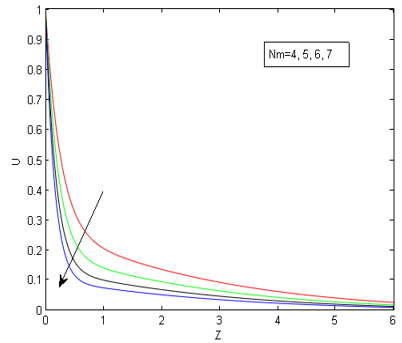Figure 6. Primary velocity versus z for Gr=5,Gm=3, Re=0.5,Da=1,Sc=816.87,N=5,Er=0.02,t=0.2, Pr=0.021,K=0.2, $\beta_{i}$=0.2, $\beta_{e}$=0.5

## 7.png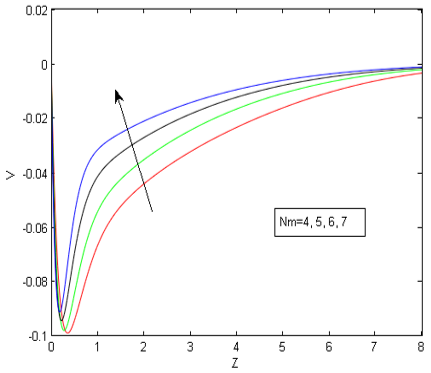Figure 7. Secondary velocity versus z for Gr=5,Gm=3, Re=0.5,Da=1,Sc=816.87,N=5,Er=0.02,t=0.2, Pr=0.021,K=0.2, $\beta_{i}$=0.2, $\beta_{e}$=0.5

## 8.pngFigure 8. Primary velocity versus z for Gr=5, Gm=3, Re=0.5, Da=1, Sc=816.87, N=5, Er=0.02, t=0.2, Pr=0.021, K=0.2, Nm=5,  $\beta_{e}$=0.5

## 9.png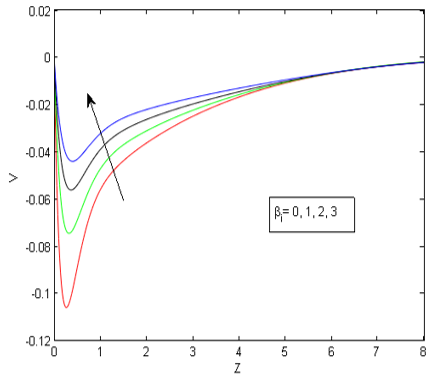Figure 9. Secondary velocity versus z for Gr=5, Gm=3, Re=0.5, Da=1, Sc=816.87, N=5, Er=0.02,t=0.2, Pr=0.021, K=0.2, Nm=5,  $\beta_{e}$=0.5

## 10.png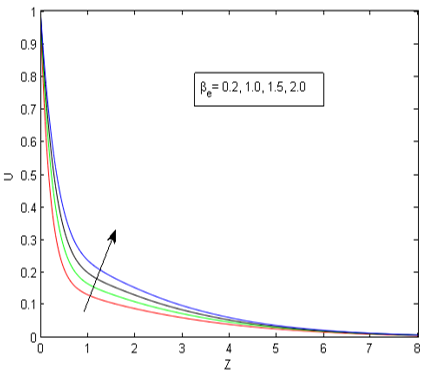Figure 10. Primary velocity versus z for Gr=5, Gm=3, Re=0.5, Da=1, Sc=816.87, N=5, Er=0.02, t=0.2, Pr=0.021, K=0.2, $\beta_{i}$=0.2, Nm=5

## 11.png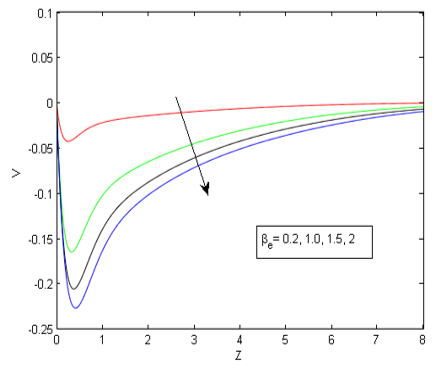Figure 11. Secondary velocity versus z for Gr=5, Gm=3, Re=0.5,Da=1,Sc=816.87,N=5, Er=0.02, t=0.2, Pr=0.021, K=0.2, $\beta_{i}$=0.2, Nm=5

## 12.pngFigure 12. Primary velocity versus z for Gr=5, Gm=3, Re=0.5, Nm=5, Sc=816.87, N=5, Er=0.02, t=0.2, Pr=0.021, K=0.2, $\beta_{i}$=0.2, $\beta_{e}$=0.5

## 13.pngFigure 13. Secondary velocity versus z for Gr=5, Gm=3, Re=0.5, Nm=5, Sc=816.87, N=5, Er=0.02, t=0.2, Pr=0.021, K=0.2, $\beta_{i}$=0.2, $\beta_{e}$=0.5

## 14.png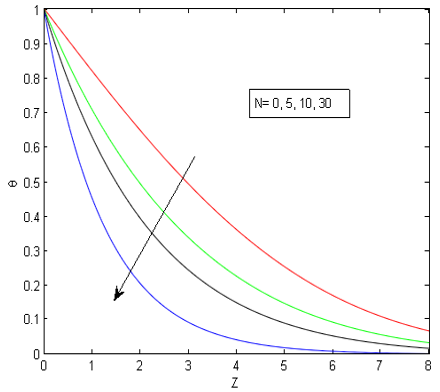Figure 14. Temperature versus z for Pr=0.021, t=0.2

## 15.pngFigure 15. Concentration versus z for Sc=816.87, t=0.2

## 16.pngFigure 16. Concentration versus z for K=0.2, t=0.2

Figures 15 and 16 represent the concentration profile versus the normal co-ordinate under the effects of chemical reaction parameter K and Schmidt number Sc respectively. Both the parameters reduce the concentration level of the fluid but their effects are realized only in a thin area adjacent to the plate. Physical justification for this behavior of fluid concentration is attributed to the very high value of Schmidt number. Very high value of Sc means very low mass diffusivity which results in a comprehensive fall in the concentration boundary layer.

Table 1. Variation of primary Cf with respect to Nm

 Nm Primary Cf 0 0.3825 0.5 0.4352 1 0.5891 1.5 0.8262 2 1.1426 2.5 1.5239 3 1.9509 3.5 2.4082 4 2.8837

Skin friction for z=0,Gr=5,Gm=3,Re=0.5,Da=1,Sc=816.87,N=5,Er=0.02,t=0.2,Pr=0.021,K=0.2, $\beta_{i}$=0.2, $\beta_{e}$=0.5

Table 2. Variation of primary Cf  and Nu with respect to N

 N Primary Cf Nu 0 3.8062 0.1828 0.5 3.8126 0.2008 1 3.8186 0.2182 1.5 3.8242 0.2351 2 3.8294 0.2514 2.5 3.8344 0.2673 3 3.8390 0.2827 3.5 3.8434 0.2977 4 3.8475 0.3123

Skin friction for z=0,Gr=5,Gm=3,Re=0.5,Da=1,Sc=816.87,Nm=5,Er=0.02,t=0.2,Pr=0.021,K=0.2, $\beta_{i}$=0.2, $\beta_{e}$=0.5

Nusselt number for z=0, Pr=0.021, t=0.2

Table 3. Variation of primary Cf  and Sh with respect to K

 K Primary Cf Sh 0 3.8557 36.0567 0.1 3.8555 36.7755 0.3 3.8551 38.1988 0.5 3.8556 39.6035 0.8 3.8565 41.6768 1 3.8570 43.0370 1.5 3.8583 46.3637 2 3.8596 49.5897 3 3.8620 55.7616

Skin friction for z=0,Gr=5,Gm=3,Re=0.5,Da=1,Sc=816.87,Nm=5,Er=0.02,t=0.2,Pr=0.021,N=5, $\beta_{i}$=0.2, $\beta_{e}$=0.5

Sherwood number for z=0, Sc=816.87, t=0.2

In Table 1, primary skin friction has been analyzed under the effect of hydro-magnetic parameter, in Table 2, primary skin friction and Nusselt number have been presented corresponding to various values of radiation parameter and in Table 3 effects of chemical reaction parameter on primary skin friction and Sherwood number have been studied. It is found that as the applied magnetic field is made stronger the primary skin friction at the plate also grows up. The radiation parameter speeds up the heat transfer from the plate to the fluid and also increases the viscous drag at the plate. Chemical reaction parameter enhances the rate of mass transfer from the plate remarkably. However, no significant variation of primary skin friction at the plate is noticed under the effect of chemical reaction parameter.

8. Conclusions

The investigation leads to the following conclusions:

-Hall parameter, ion-slip parameter and Darcy parameter accelerate and radiation parameter, rotation parameter and hydromagnetic parameter decelerate the primary velocity. On the contrary, secondary velocity is accelerated by the rotation parameter and decelerated by the ion-slip parameter.

-Temperature decreases with radiation parameter.

-Chemical reaction parameter and Schmidt number reduce the concentration level but the effects are only limited to a thin area adjacent to the plate.

-Magnetic parameter and radiation parameter increase the primary skin friction at the plate.

-Heat transfer becomes faster under the effect of radiation parameter and chemical reaction parameter enhances the rate of mass transfer.

Nomenclature
 $\vec{B}$ Magnetic induction vector ${{B}_{0}}$ Magnitude of applied magnetic field ${C}'$ Fluid concentration ${{C}_{f}}$ Skin friction coefficient ${{C}_{P}}$ Specific heat at constant pressure $Da$ Darcy number ${{D}_{M}}$ Mass diffusivity ${{E}_{r}}$ Rotational parameter $\vec{g}$ Acceleration due to gravity $\text{Gm}$ Solutal Grashof number $\text{Gr}$ Grashof number $\vec{J}$ Current density vector $K$ Chemical reaction parameter ${{K}_{1}}$ Chemical reaction constant ${{K}_{p}}$ Permeability parameter ${{K}_{p}}^{*}$ Permeability constant $L$ Characteristic length $N$ Radiation parameter $Nm$ Hydromagnetic parameter $\text{Nu}$ Nusselt number $p$ Fluid pressure $\text{Pr}$ Prandtl number $\vec{q}$ Fluid velocity Sc Schmidt number Sh Sherwood number $t^{\prime}$ Time $T^{\prime}$ Fluid temperature Greek symbols $\beta$ Volume expansion coefficient for heat transfer ${{\beta }^{*}}$ Volume expansion coefficient for mass transfer ${{\beta }_{e}}$ Hall parameter ${{\beta }_{i}}$ Ion slip parameter $\kappa$ Thermal conductivity $\lambda$ Wave length ${{\left( {{a}_{\lambda }} \right)}_{w}}$ Absorption coefficient at the plate ${{e}_{\lambda h}}$ Planck’s function $\mu$ Coefficient of viscosity $\nu$ Kinematic viscosity $\rho$ Fluid density $\sigma$ Electrical conductivity $\vec{\omega }$ Angular velocity
Appendix

$\left(H_{0}\right)^{2}=(N m)^{2} \alpha_{0} M_{1}=2 i E r+\left(H_{0}\right)^{2}$

$a=M_{1}+\frac{1}{D a(\mathrm{Re})^{2}} B_{1}=\frac{\mathrm{Gr}}{\operatorname{Pr}-1} D_{1}=\frac{a-\operatorname{Pr} N}{\operatorname{Pr}-1}$

$E_{1}=\frac{\mathrm{Gm}}{\mathrm{Sc}-1} \quad F_{1}=\frac{a-\mathrm{Sc} K}{\mathrm{Sc}-1}$

$\psi(\xi, \eta, y, t)=\frac{1}{2}\left[e^{\sqrt{\zeta \eta} y} \operatorname{erfc}\left(\frac{\sqrt{\xi} y}{2 \sqrt{t}}+\sqrt{\eta t}\right)+e^{-\sqrt{\xi \eta} y} \operatorname{erfc}\left(\frac{\sqrt{\xi} y}{2 \sqrt{t}}-\sqrt{\eta t}\right)\right]$

$\psi^{\prime}(\xi, \eta, t)=\sqrt{\xi \eta} \operatorname{erf}(\sqrt{\eta t})+e^{-\eta t} \sqrt{\frac{\xi}{\pi t}}$

References

 Cowling, T.G. (1957). Magnetohydrodynamics. Wiley Inter Science, New York.

 Pop, I. (1971). The effect of Hall currents on hydromagnetic flow near an accelerated plate. Journal of Mathematical and Physical Sciences, 5: 375-379.

 Cramer, K.R., Pai, S.I. (1973). Magneto Fluid Dynamics for Engineers and Applied Physicists. McGraw Hill, New York, USA.

 Ahmed, N., Sarmah, H.K. (2011). MHD transient flow past an impulsively started infinite horizontal porous plate in rotating system with Hall current. Int. J. of Appl. Math. And Mech, 7(2): 1-15.

 Seth, G.S., Mahato, G.K., Sarkar, S. (2013). Effects of Hall current and rotation on MHD natural convection flow past an impulsively moving vertical plate with ramped temperature in the presence of thermal diffusion with heat absorption. International Journal of Energy \& Technology, 5(16): 1-12.

 Seth, G.S., Kumbhakar, B., Sarkar, S. (2017). Unsteady MHD natural convection flow with exponentially accelerated free-stream past a vertical plate in the presence of Hall current and rotation. Rendiconti del Circolo Matematico di Palermo Series 2, 66(3): 263-283. https://doi.org/10.1007/s12215-016-0250-1

 Mohanty, H.K. (1977). Hydromagnetic Rayleigh problem with Hall effect. Czechoslovak Journal of Physics, 27(10): 1111-1116. https://doi.org/10.1007/BF01588999

 Soundalgekar, V.M., Vighnesam, N.V., Takhar, H.S. (1979). Hall and ion-slip effects in MHD Couette flow with heat transfer. IEEE Transactions on Plasma Science, 7(3): 178-182. https://doi.org/10.1109/TPS.1979.4317226

 Ghosh, S.K., Beg, O.A., Rawat, S., Beg, T.A. (2009). Three dimensional rotating hydromagnetic transient convective flow of liquid metal in porous medium with Hall and Ion-slip current effects. Emirates Journal of Engineering Research, 14(2): 45-57.

 Sheikh, A.H., Ahmed, N. (2016). Radiation effect on three dimensional rotating MHD unsteady convective flow of liquid metal in a porous medium in presence of Hall and Ion-slip current. Journal of Energy, Heat and Mass Transfer, 38: 1-23.

 Eckert, E.R., Drake, R.M. (1972). Analysis of Heat and Mass Transfer. Mc-Graw Hill, NY.

 Raptis, A. (1983). MHD natural convection and mass transfer through a horizontal porous channel. Acta Physica Hungarica, 54(1): 213-215. https://doi.org/10.1007/BF03158705

 Acharya, M., Dash, G.C., Singh, L.P. (2000). Magnetic field effects on the free convection and mass transfer flow through porous medium with constant suction and constant heat flux. Indian Journal of Pure & Applied Mathematics, 31(1): 1-18.

 Reddy, G.V.R., Murthy, C.V.R., Reddy, N.B. (2011). Unsteady MHD free convective mass transfer flow past an infinite vertical porous plate with variable suction and Soret effect. Int. J. Appl. Maths. Mech., 7(21): 70-84. http://hdl.handle.net/20.500.11948/946

 Lavanya, B., Leela Ratnam, A. (2014). Radiation and mass transfer effects on unsteady MHD free convective flow past a vertical porous plate embedded in a porous medium in a slip flow regime with heat source/sink and Soret effect. IJETR, 2: 210-220.

 Sherman, A., Sutton, G.W. (1965). Engineering Magnetohydrodynamics. McGraw-Hill, New York.